JEE  >  Test: Previous Year Questions: States of Matter

# Test: Previous Year Questions: States of Matter

Test Description

## 14 Questions MCQ Test Chemistry Class 11 | Test: Previous Year Questions: States of Matter

Test: Previous Year Questions: States of Matter for JEE 2023 is part of Chemistry Class 11 preparation. The Test: Previous Year Questions: States of Matter questions and answers have been prepared according to the JEE exam syllabus.The Test: Previous Year Questions: States of Matter MCQs are made for JEE 2023 Exam. Find important definitions, questions, notes, meanings, examples, exercises, MCQs and online tests for Test: Previous Year Questions: States of Matter below.
Solutions of Test: Previous Year Questions: States of Matter questions in English are available as part of our Chemistry Class 11 for JEE & Test: Previous Year Questions: States of Matter solutions in Hindi for Chemistry Class 11 course. Download more important topics, notes, lectures and mock test series for JEE Exam by signing up for free. Attempt Test: Previous Year Questions: States of Matter | 14 questions in 14 minutes | Mock test for JEE preparation | Free important questions MCQ to study Chemistry Class 11 for JEE Exam | Download free PDF with solutions
 1 Crore+ students have signed up on EduRev. Have you?
Test: Previous Year Questions: States of Matter - Question 1

### According to the kinetic theory of gases, in an ideal gas, between two successive collisions a gas molecule travels –    [AIEEE-2003]

Detailed Solution for Test: Previous Year Questions: States of Matter - Question 1

According to kinetic theory the gas molecules are in a state of constant rapid motion in all possible directions colloiding in a random manner with one another and with the walls of the container and between two successive collisions molecules travel in a straight line path but show haphazard motion due to collisions.

Test: Previous Year Questions: States of Matter - Question 2

### As the temperature is raised from 20°C to 40°C, the average kinetic energy of neon atoms changes by a factor of which of the following ? [AIEEE-2004]

Detailed Solution for Test: Previous Year Questions: States of Matter - Question 2

Average kinetic energy ∝ Temperature in Kelvin

(KE40) / (KE20) = (3/2 nR * 313)/(3/2 nR*293)

KE40 / KE20 = T2/T1 = 40 + 273 / 20 + 273  = 313/293

Test: Previous Year Questions: States of Matter - Question 3

### In van der Waals equation of state of the gas law, the constant `b' is a measure of - [AIEEE-2004]

Detailed Solution for Test: Previous Year Questions: States of Matter - Question 3

The correct answer is Option C.

In van der Waals equation of state of the gas law, the constant b is a measure of the volume occupied by the molecules.

It gives the effective size of the gas molecules. The greater value of b indicates a larger size of the molecules and smaller compressible volume.

Test: Previous Year Questions: States of Matter - Question 4

Which one of the following statements is NOT true about the effect of an increase in temperature on the distribution of molecular speeds in a gas ?

[AIEEE-2005]

Detailed Solution for Test: Previous Year Questions: States of Matter - Question 4

Most probable velocity increase and fraction of molecule possessing most probable velocity decrease.

Test: Previous Year Questions: States of Matter - Question 5

Equal masses of methane and oxygen are mixed in an empty container at 25ºC. The fraction of the total pressure exerted by oxygen is –

[AIEEE-2007]

Detailed Solution for Test: Previous Year Questions: States of Matter - Question 5

Let the mass of methane and oxygen be m gm.
Mole fraction of oxygen, xO2 = m/32/(m/32 +m/16) = 1/3
Let the total pressure be P.
Partial pressure of O2, pO2 = P × xO2 = P x 1/3 = P/3

Test: Previous Year Questions: States of Matter - Question 6

The no. of moles per litre in the equation PV = nRT is expressed by -

[AIEEE-2002]

Detailed Solution for Test: Previous Year Questions: States of Matter - Question 6

Number of moles per litre can be represented as n/V so if we shift RT to the left side and V to the right side then we can get n/V that is our number of moles per litre is equal to p/RT and so option a is correct.

Test: Previous Year Questions: States of Matter - Question 7

The correct value of R is -

[AIEEE-2002]

Detailed Solution for Test: Previous Year Questions: States of Matter - Question 7

The different values of R are as follow:-
8.314 J mol-1 K-1, 8.314×107 erg mol-1 K-1, 0.0821 atm-lit mol-1 K-1 or 2 cal mol-1 K-1

Test: Previous Year Questions: States of Matter - Question 8

If 10-4 dm3 of water is introduced into a 1.0 dm3 flask at 300 K, how many moles of water are in in the vapour phase when equilibrium is established ?

(Given : Vapour pressure of H2O at 300 is 3170 pa; R = 8.314 JK-1 mol)

[AIEEE -2010]

Detailed Solution for Test: Previous Year Questions: States of Matter - Question 8

The volume occupied by water molecules in vapour phase is (1×10−4) dm3, that is approximately (1×10−3) m3.
pvapV = nH2O mol
3170 × 1 × 10−3 = nH2O × 8.314 × 300K
nH2O = 3170 × 1 × 10−3 / 8.314 × 300
= 1.27 × 10−3 mol

Test: Previous Year Questions: States of Matter - Question 9

`a' and `b' are Vander Waals' constant for gases. Chlorine is more easily liquefied than ethane because :

[AIEEE - 2011]

Detailed Solution for Test: Previous Year Questions: States of Matter - Question 9

a and b are the van der Waals constant for gases where ‘a’ means the force of attraction while ‘b’ is the effective size of the molecule. Chlorine is more easily liquefied than Ethane so ‘a’  of chlorine will must be greater than a value of Ethane. Also, Chlorine is more easily liquefied then the ‘b’ of chlorine should be less than ‘b’ of Ethane. For smaller size we have more attraction and so the liquefaction will be easy.

Test: Previous Year Questions: States of Matter - Question 10

When r, P and M represent rate of diffusion, pressure and molecular mass, respectively, then the ratio of the rates of diffusion (rA/rB) of two gases A and B, is given as -

[AIEEE - 2011]

Detailed Solution for Test: Previous Year Questions: States of Matter - Question 10

The correct answer is option A
Rate of diffusion: r     α    p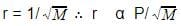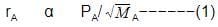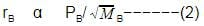rA/rB = pA/pB.(MB/MA)1/2
Hence, pBpA (MB/MA)1/2 is the answer.

Test: Previous Year Questions: States of Matter - Question 11

The molecular velocity of any gas is -

[AIEEE - 2011]

Detailed Solution for Test: Previous Year Questions: States of Matter - Question 11

The average kinetic energy of a gas particle is directly proportional to the temperature. An increase in temperature increases the speed in which the gas molecules move. All gases at a given temperature have the same average kinetic energy. Lighter gas molecules move faster than heavier molecules.

Test: Previous Year Questions: States of Matter - Question 12

The compressibility factor for a real gas at high pressure is –

[AIEEE-2012]

Detailed Solution for Test: Previous Year Questions: States of Matter - Question 12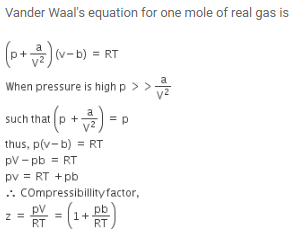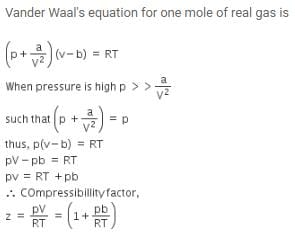Test: Previous Year Questions: States of Matter - Question 13

For gaseous state, if most probable speed is denoted by C*, average speed by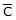and mean square speed by C, then for a large number of molecules the ratios of these speeds are :

[Jee(Main) 2013, 3/120]

Detailed Solution for Test: Previous Year Questions: States of Matter - Question 13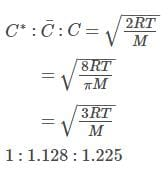Test: Previous Year Questions: States of Matter - Question 14

A gaseous hydrocarbon gives upon combustion 0.72 g of water and 3.08 g. of CO2. The empirical formula of the Hydrocarbon is :

[Jee(Main) 2013, 3/120]

Detailed Solution for Test: Previous Year Questions: States of Matter - Question 14

General equation for combustion of hydrocarbon:
CxHy + (x+ y/4)O2 → xCO2 + (y/2)H2O
Number of moles of CO2 produced = 3.08/44 = 0.07
Number of moles of H2O produced = 0.72/18 = 0.04
SO, x / (y/2) = 0.07/0.04 =  7/4
The formula of hydrocarbon is C7H8

## Chemistry Class 11

204 videos|331 docs|229 tests
 Use Code STAYHOME200 and get INR 200 additional OFF Use Coupon Code
Information about Test: Previous Year Questions: States of Matter Page
In this test you can find the Exam questions for Test: Previous Year Questions: States of Matter solved & explained in the simplest way possible. Besides giving Questions and answers for Test: Previous Year Questions: States of Matter, EduRev gives you an ample number of Online tests for practice

## Chemistry Class 11

204 videos|331 docs|229 tests